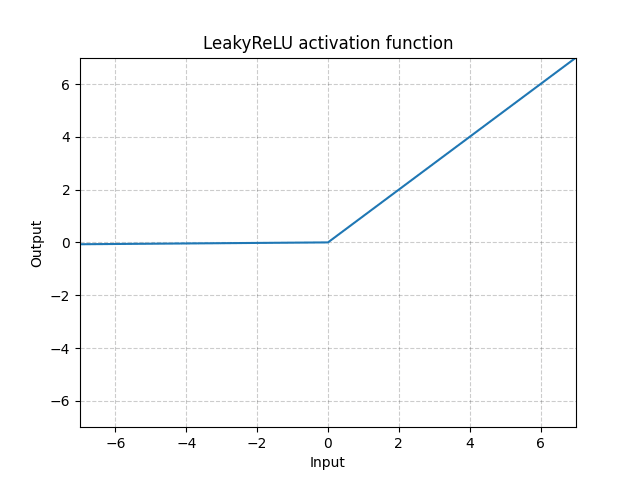/PyTorch

# LeakyReLU

class torch.nn.LeakyReLU(negative_slope: float = 0.01, inplace: bool = False) [source]

Applies the element-wise function:

$\text{LeakyReLU}(x) = \max(0, x) + \text{negative\_slope} * \min(0, x)$

or

$\text{LeakyRELU}(x) = \begin{cases} x, & \text{ if } x \geq 0 \\ \text{negative\_slope} \times x, & \text{ otherwise } \end{cases}$
Parameters
• negative_slope – Controls the angle of the negative slope. Default: 1e-2
• inplace – can optionally do the operation in-place. Default: False
Shape:
• Input: $(N, *)$ where * means, any number of additional dimensions
• Output: $(N, *)$ , same shape as the inputExamples:

>>> m = nn.LeakyReLU(0.1)
>>> input = torch.randn(2)
>>> output = m(input)


© 2019 Torch Contributors
Licensed under the 3-clause BSD License.
https://pytorch.org/docs/1.7.0/generated/torch.nn.LeakyReLU.html